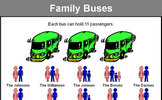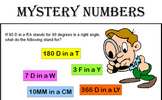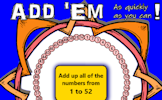# Ratio and scale

### Term 1 starting in week 1 :: Estimated time: 2 weeks

• Understand the meaning and representation of ratio
• Understand and use ratio notation
• Solve problems involving ratios of the form 1:n (or n:1)
• Solve proportional problems involving the ratio m:n
• Divide a value into a given ratio
• Express ratios in ther simplest integer form
• Express ratios in the form 1:n
• Compare ratios and related fractions
• Understand IT as the ratio between diameter and circumference
• Understand gradient of a line as a ratio

This page should remember your ticks from one visit to the next for a period of time. It does this by using Local Storage so the information is saved only on the computer you are working on right now.

## Lesson Starters

Here are some suggestions for whole-class, projectable resources which can be used at the beginnings of each lesson in this block.

### 1st Lesson#### Aunty Dotty

A real life situation requiring the division an amount of money according to a given ratio.

### 2nd Lesson#### Big Bieber

If the dimensions of an object double, its volume increases by a factor of eight.

### 3rd Lesson#### Family Buses

Fit families onto eleven seater buses without splitting up the families.

### 4th Lesson#### Four Problems

For mathematical questions to get everyone thinking at the beginning of the lesson.

### 5th Lesson#### Mystery Numbers

Can you recognise the mystery numbers from the clues?

### 6th LessonAdd up a sequence of consecutive numbers. Can you find a quick way to do it?

Some of the Starters above are to reinforce concepts learnt, others are to introduce new ideas while others are on unrelated topics designed for retrieval practice or and opportunity to develop problem-solving skills.

White Rose ResourcesEnd of block assessments provide a quick progress check at the end of each block of learning to make sure students have understood the content covered. This Scheme of Learning was produced by White Rose Maths and is used here with permission granted on 30th June 2021.For All: